Courses

# Electromagnetic Induction MSQ

## 10 Questions MCQ Test Topic wise Tests for IIT JAM Physics | Electromagnetic Induction MSQ

Description
This mock test of Electromagnetic Induction MSQ for Physics helps you for every Physics entrance exam. This contains 10 Multiple Choice Questions for Physics Electromagnetic Induction MSQ (mcq) to study with solutions a complete question bank. The solved questions answers in this Electromagnetic Induction MSQ quiz give you a good mix of easy questions and tough questions. Physics students definitely take this Electromagnetic Induction MSQ exercise for a better result in the exam. You can find other Electromagnetic Induction MSQ extra questions, long questions & short questions for Physics on EduRev as well by searching above.
*Multiple options can be correct
QUESTION: 1

### Switch S  is closed for a long time at  t = 0. It is opened, then which of the following statement is incorrect.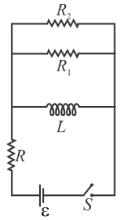Solution:

Just before opening the switch, the current in the inductor is ε/R. Energy stored in it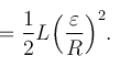This energy will dissipate in the resistors R1  and  R2  in the ratio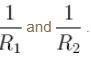The correct answers are: total heat produced in resistor R  after opening the switch is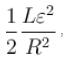total heat produced in resistor R1  after opening the switch is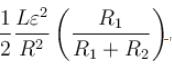no heat will be produced in  R1

*Multiple options can be correct
QUESTION: 2

### A constant force F is being applied on a rod of length ‘l’  kept at rest on two parallel conducting rails connected at ends by resistance R in uniform magnetic field B  as shown. Which of the following statement is incorrect.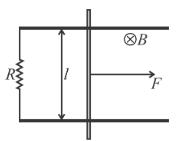Solution: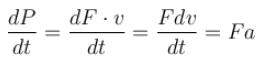as ‘a’  is decreasing continuously hence the rate of power delivered by external force will be decreasing continuously.

The correct answers are: the power delivered by force will be constant with time, the power delivered by force will be increasing first and then will decreases, the rate of power delivered by the external force will be increasing continuously

*Multiple options can be correct
QUESTION: 3

### In the figure shown ‘R’  is a fixed conducting fixed ring of negligible resistance and radius ‘a’. PQ  is a uniform rod of resistance r. It is hinged at the centre of the ring and rotated about this point in clockwise direction with a uniform angular velocity ω. There is a uniform magnetic field of strength ‘B’  pointing inwards ‘r’  is a stationary resistance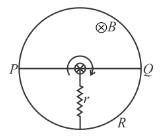Solution:

Equivalent circuit :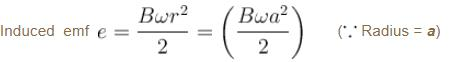By nodal equation :

nodal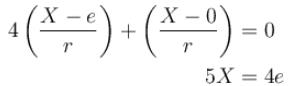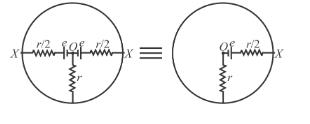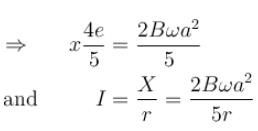also direction of current in ‘r’ will be towards negative terminal i.e. from rim to origin.
Alternatively; by equivalent of cells (figure (ii)):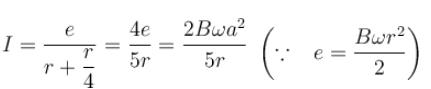The correct answers are: Current through ‘r’  is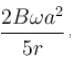Direction of current in external ‘r’  is from circumference to centre

*Multiple options can be correct
QUESTION: 4

In the figure shown, the magnet is pushed towards the fixed ring along the axis of the ring and it passes through the ring. Which of the following is incorrect.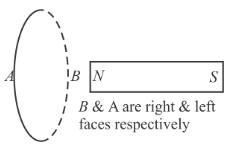Solution:

When the magnetic goes away from the ring the flux in the ring decreases hence the induced current will be such that it opposes the decreasing flux in it hence ring will behave like a magnet having face A  as north pole and face B  as south pole.

The correct answers are: when magnet goes towards the ring the face B becomes south pole and the face A becomes north pole, when magnet goes away from the ring the face B becomes north pole and the face A becomes south pole, the face A will always be a north pole.

*Multiple options can be correct
QUESTION: 5

In the circuit diagram shown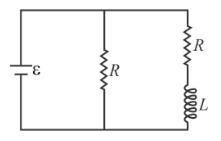Solution:

At steady state no resistance will be offered by inductor hence, time constant is L/R  and steady state current in inductor is ε/R.

The correct answers are: time constant is  L/R , steady state current in inductor is  ε/R

*Multiple options can be correct
QUESTION: 6

An ideal inductor, (having initial current zero) a resistor and an ideal battery are connected in series at time t = 0. At any time t, the battery supplies energy at the rate PB, the resistor dissipates energy at the rate PR and the inductor stores energy at the rate PL.

Solution:

By principal of energy conservation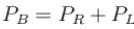Near the starting of the circuit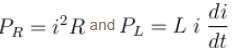As di/dt has greater value at the starting of the circuit,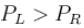The correct answers are: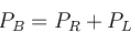for all times t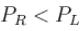only near the starting of the circuit

*Multiple options can be correct
QUESTION: 7

Two straight conducting rails form a right angle where their ends are joined. A conducting bar in contact with the rails starts at the vertex at time t = 0 and moves with constant velocity v along them as shown in figure. A magnetic field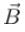is directed into the page. The induced emf  in the circuit at any time t  is proportional to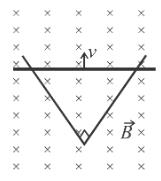Solution: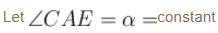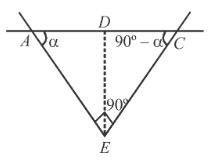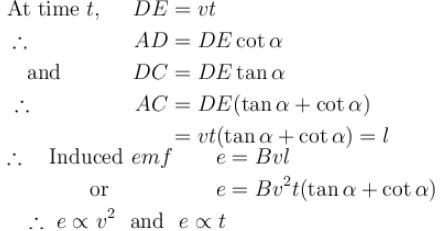*Multiple options can be correct
QUESTION: 8

A conducting rod of length l is moved at constant velocity v0 on two parallel, conducting, smooth, fixed rails, that are placed in a uniform constant magnetic field B perpendicular to the plane of the rails as shown in figure. A resistance R is connected between the two ends of the rail. Then which of the following is/are correct: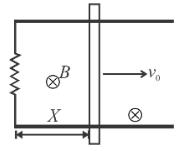Solution:

Rate of work done by external agent is :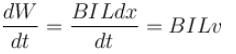and thermal power dissipated in the resistor = eI = (BvL)I

clearly both are equal.

If applied external force is doubled, the rod will experience a net force and hence acceleration. As a result velocity increases.
Since I = e/R

On doubling ‘R’, current and hence required power becomes half.

Since, P = BILv

The correct answers are: The thermal power dissipated in the resistor is equal to rate of work done by external person pulling the rod, If applied external force is doubled than a part of external power increases the velocity of rod, If resistance R is doubled then power required to maintain the constant velocity v0 becomes half

*Multiple options can be correct
QUESTION: 9

A circuit consisting of a constant emf‘E’, a self induction ‘L’ and a resistance ‘R’  is closed at  t = 0. The relation between the current I in the circuit and time t  is as shown by curve ‘a’  in the figure. When one or more of parameters E, R and L are changed, the curve ‘b’ is obtained. The steady state current is same in both the cases. Then it is possible that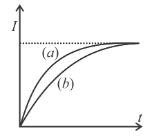Solution:

Since curve ‘b’  is below curve ‘a’  hence it is possible that   and  R  are kept constant and L is increased or  E  and R are both halved and  L  is kept constant.

The correct answers are: E and are kept constant and L is increased , E  and R are both halved and L is kept constant

*Multiple options can be correct
QUESTION: 10

A conducting loop rotates with constant angular velocity about its fixed diameter in a uniform magnetic field. Whose direction is perpendicular to that fixed diameter.

Solution: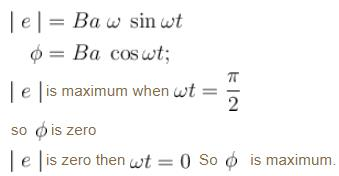The correct answers are: The emf  will be maximum at the moment when flux is zero, The emf  will be zero at the moment when flux is maximum, The emf  will be maximum at the moment when plane of the loop is parallel to the magnetic field, The phase difference between the flux and the emf  is π/2